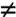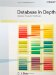# Evaluating SQL Expressions

 In addition to natural join, Codd originally defined an operator he called theta-join, where theta denoted any of the usual scalar comparison operators ("=", "", "<", and so on). The operator isnt primitive; in fact, it's equivalent to a restriction of a cartesian product. Here by way of example is a Tutorial D formulation of the "not equals"-join of suppliers and parts on cities (so theta here is ""): ` ( ( S RENAME ( CITY AS SCITY ) TIMES ( P RENAME ( CITY AS PCITY ) ) WHERE SCITYPCITY ` Note that the result has two "city" attributes, SCITY and PCITY. An SQL analog: ` SELECT S.SNO, S.SNAME, S.STATUS, S.CITY AS SCITY, P.PNO, P.PNAME, P.COLOR, P.WEIGHT, P.CITY AS PCITY FROM S, P WHERE S.CITY <> P.CITY ` We can think of this SQL expression as being implemented in three steps, as follows: The FROM clause is executed and yields the cartesian product of tables S and P. (If we were doing this relationally, we would have to rename the city attributes before that cartesian product is computed. SQL gets away with renaming them afterward because its tables have a left-to-right ordering to their columns, meaning it can distinguish the two city columns by their ordinal position. For simplicity, let's ignore this detail.)Next, the WHERE clause is executed and yields a restriction of that cartesian product by eliminating rows in which the two city values are equal.[*][*] If theta had been "=" instead of "", Step 2 would have been: Restrict the cartesian product by equijoin of suppliers and parts on cities. In other words, an equijoin is a theta-join for which theta is "=". Exercise: What's the difference between an equijoin and a natural join?Finally, the SELECT clause is executed and yields a projection of that restriction on the columns specified in the SELECT clause. (Of course, the SELECT clause is doing some renaming as well in this particular example and later we'll see that it also provides functionality similar to that of the relational extend operator. What's more, it generally doesn't eliminate duplicates, unless DISTINCT is specified. But I want to ignore all of these details too, for simplicity.) At least to a first approximation, then, the FROM clause corresponds to a cartesian product, the WHERE clause to a restriction, and the SELECT clause to a projection, and the overall SELECT - FROM - WHERE expression represents a projection of a restriction of a cartesian product. It follows that I've just given a loose, but reasonably formal, definition of the semantics of SQL's SELECT - FROM - WHERE expressions; equivalently, I've given a conceptual algorithm for evaluating such expressions. Of course, there's no implication that the implementation has to use exactly that algorithm in order to implement such expressions; au contraire, it can use any algorithm it likes, just so long as whatever algorithm it does use is guaranteed to give the same result as the conceptual one. And there are often good reasons usually performance reasons for using a different algorithm, thereby (for example) executing the clauses in a different order or otherwise rewriting the original query. However, the implementation is free to do such things only if it can be proved that the algorithm it does use is logically equivalent to the conceptual one. Indeed, one way to characterize the job of the system optimizer is to find an algorithm that's guaranteed to be equivalent to the conceptual one but performs better.Database in Depth: Relational Theory for Practitioners
ISBN: 0596100124
EAN: 2147483647
Year: 2006
Pages: 127
Authors: C.J. Date

Similar book on Amazon